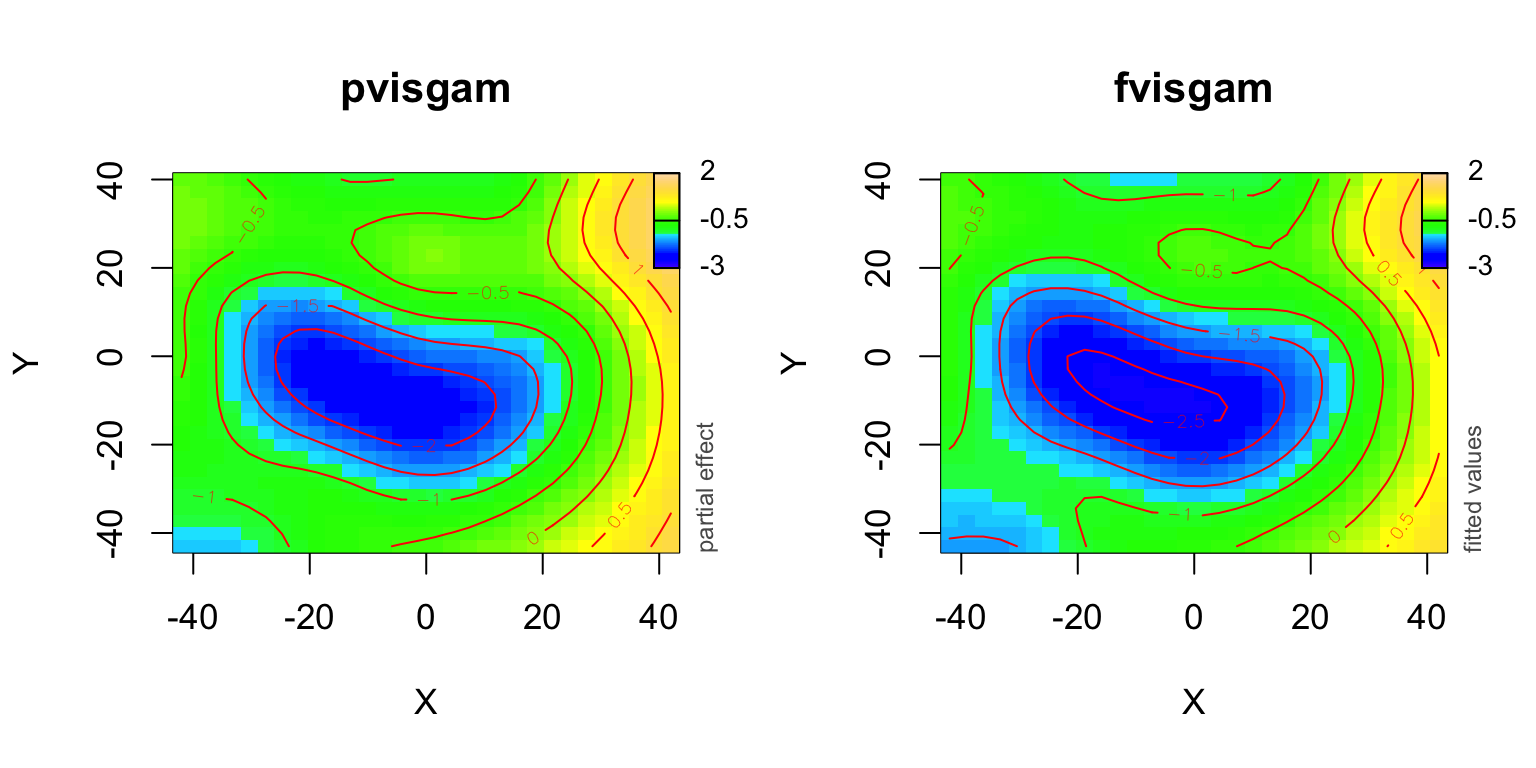# Packages & data

For creating animations you need to install the package `animation` from CRAN:

``````# when being asked for a mirrir to choose,
# you could choose the first one:
install.packages("animation")``````

For this example you will need the newest version of the package `itsadug`, at least version 2.2 (on CRAN) or newer versions, see my website:

``install.packages("itsadug", repos="http://cran.us.r-project.org")``

``````library(animation)
library(mgcv)
# set info messages to true:
infoMessages("on")``````

For the EEG example I used the data of one participant of the Kryuchkova et al. (2012) study. Note that this example is only for illustration purposes and does not present the best analysis. You can download a simplified version of the data here or run the following code:

``download.file("http://jacolienvanrij.com/Tutorials/Files/EEG_example.rds", "EEG_example.rds", mode="wb")``

``````dat <- readRDS("EEG_example.rds")

``````##   Electrode Item Trial      Time Animate      Amp   X  Y
## 1       AF3   w1   116 -192.1788     yes 9.312411 -16 31
## 2       AF3   w1   116 -184.3575     yes 6.989169 -16 31
## 3       AF3   w1   116 -176.5363     yes 7.051056 -16 31``````

# Example: A GAMM model to investigate the pattern over different electrodes

Generalized Additive Mixed Modeling (GAMM; see Wood (2006) for more info) is a nonlinear regression technique that allows to investigate the changes in voltages measured by different electrodes over time.

We run a relatively simple nonlinear regression model that only includes `Time` and an interaction between `X` (approximate position of the electrode on the scalp, determining on which hemisphere the electrode is located) and `Y` (approximate position of the electrode on the scalp, determining how frontal / backward the electrode is located).

``````# may take some time to run:
m1<- bam(Amp ~ te(Time, X, Y, k=c(20,5,5), d=c(1,2)),
data=dat, discrete=TRUE)``````
We can inspect the model by printing the summary and plotting the modelterms using the following R code. Note: In R markdown add ````{r, results='asis'}` on top of the R block to show the output as HTML table. In addition, some HTML code was included in the markdown file to increase the padding of the table cells: `<style>td { padding:2pt 10pt;}</style>`
``gamtabs(m1, type='HTML')``
 A. parametric coefficients Estimate Std. Error t-value p-value (Intercept) -0.2208 0.0305 -7.2395 < 0.0001 B. smooth terms edf Ref.df F-value p-value te(Time,X,Y) 100.1025 113.7483 28.6043 < 0.0001

In the package `mgcv` that implements GAMMs interaction surfaces are by default plotted using a contour plot generated by the function `plot`. However, a three-way interaction is not possible (i.e., `plot(m1, select=1)` will generate an error). Instead, we could plot the partial effect of the interaction with `pvisgam()`, which uses colors to improve the readability (left plot below).

``pvisgam(m1, view=c("X","Y"), zlim=c(-3,2))``

Alternatively, we could use the function `fvisgam()`. In contrast with `pvisgam`, the function `fvisgam` visualizes the fitted effects. In other words, all modelterms (`Intercept`, `s(Time)` and `s(X,Y)`) are summed together and are plotted (right plot below). As the intercept is close to 0 here, the functions do not result in very different plots.

Note that in both plots the predictor `Time` is automatically set to the value 50, but we can change this value with the argument `cond`.

``````# Note the scale in z-scale:
fvisgam(m1, view=c("X","Y"), cond=list(Time=150), zlim=c(-3,2))``````With the function `plot_topo` we could visualize the electrode positions on the scalp. This function requires the electrode positions as a list with ‘X’ and ‘Y’ coordinates, which we could retrieve from the data:

``````electrodes <- dat[,c("X","Y", "Electrode")]
electrodes <- as.list(electrodes[!duplicated(electrodes),])
str(electrodes)``````
``````## List of 3
##  \$ X        : int [1:32] -16 16 -21 21 -10 10 -30 30 0 -18 ...
##  \$ Y        : int [1:32] 31 31 0 0 -11 -11 -12 -12 0 22 ...
##  \$ Electrode: chr [1:32] "AF3" "AF4" "C3" "C4" ...``````

The function `topo_plot` calls the functions `pvisgam`, `fvisgam` or `plot_diff2`, so their arguments need to be provided. Make sure to make the size of the plot regions squared.

``````par(mfrow=c(1,2), cex=1.1)

plot_topo(m1, view=c("X","Y"), fun='pvisgam', zlim=c(-3,2),
el.pos=electrodes, cond=list(Time=150),
main='pvisgam')
# automatically the function fvisgam is being selected:
plot_topo(m1, view=c("X","Y"), zlim=c(-3,2),
el.pos=electrodes, cond=list(Time=150),
main='fvisgam')``````